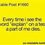# SMO 2015 Q10 Round 1

When a polynomial $f(x)$ is divided by $(x - 1)$and $(x + 5)$, the remainders are -6 and 6 respectively. Let $r(x)$ be the remainder when $f(x)$ is divided by $x^2 +4x - 5$. Find the value of $r(-2)$.

(A) 0 (B) 1 (c) 2 (D) 3 (E) 5

How does one do this sort of question?

I'm not experienced with polynomials, so it may seem a simple question to you but not to me. But please help! I'm a learner too :)Note by Timothy Wan
5 years, 6 months ago

This discussion board is a place to discuss our Daily Challenges and the math and science related to those challenges. Explanations are more than just a solution — they should explain the steps and thinking strategies that you used to obtain the solution. Comments should further the discussion of math and science.

When posting on Brilliant:

• Use the emojis to react to an explanation, whether you're congratulating a job well done , or just really confused .
• Ask specific questions about the challenge or the steps in somebody's explanation. Well-posed questions can add a lot to the discussion, but posting "I don't understand!" doesn't help anyone.
• Try to contribute something new to the discussion, whether it is an extension, generalization or other idea related to the challenge.

MarkdownAppears as
*italics* or _italics_ italics
**bold** or __bold__ bold
- bulleted- list
• bulleted
• list
1. numbered2. list
1. numbered
2. list
Note: you must add a full line of space before and after lists for them to show up correctly
paragraph 1paragraph 2

paragraph 1

paragraph 2

[example link](https://brilliant.org)example link
> This is a quote
This is a quote
    # I indented these lines
# 4 spaces, and now they show
# up as a code block.

print "hello world"
# I indented these lines
# 4 spaces, and now they show
# up as a code block.

print "hello world"
MathAppears as
Remember to wrap math in $$ ... $$ or $ ... $ to ensure proper formatting.
2 \times 3 $2 \times 3$
2^{34} $2^{34}$
a_{i-1} $a_{i-1}$
\frac{2}{3} $\frac{2}{3}$
\sqrt{2} $\sqrt{2}$
\sum_{i=1}^3 $\sum_{i=1}^3$
\sin \theta $\sin \theta$
\boxed{123} $\boxed{123}$

Sort by:

What is $r$ in $x^2+4r-5$?

- 5 years, 6 months ago

It's an error. Just corrected the note. Sorry!

- 5 years, 6 months ago

Have you read the remainder factor theorem? If so, how do you think this could apply?

Staff - 5 years, 6 months ago

yeah you are right, but here, we would apply more of Euclids Division Algorithm for polynomials. Won't we? (Which essentially constitutes the proof of remainder theorem)

- 5 years, 6 months ago

There are many equivalent ways of expressing the ideas involved in this question. I was pointing out one possible approach, which I think should be thought of given the phrasing of the question.

Staff - 5 years, 6 months ago

Since the divisor is of degree 2, $r(x)$ is linear and in the form $Ax+B$.

$f(1)=A(1)+B=A+B=-6$.

Also $f(-5)=A(-5)+B=-5A+B=6$.

Solving the simultaneous equations, $A=-2,B=-4$

$r(x)=-2x-4$

$\therefore r(-2)=-2(-2)-4=0$

Is this a correct solution?

- 5 years, 6 months ago

You got confused between $f(x)$ and $r(x)$.

- 5 years, 6 months ago